### Patterns and symmetry - Worksheets

CBSE Worksheet-01

Class - IV Mathematics – Patterns and symmetry

Time Allowed: 30 minutes

1. Fill in the blanks with suitable words:
1. Complete the sequence: 36, 45, ____, 63

2. Complete the pattern : 44, 48, 52, 56, ____, ____

3. 55,  66, 77, ____, 99

4. Start from 50 and subtract 5 each time to fill in the blanks: ______, ______, ______, _____

5. The next number completes the sequence 25, 20, 15, 10,  ________ .

2. State whether each of the following statement is True or False:
1. Pattern 2, 12, 22, 32 is starting with 2 and follow the "add 10" rule.

2. 9th term of pattern 10, 20, 30, 40 is 90.

3. Symmetrical objects are identical to each other.

4. Pattern 10,15, 20, 25 is starting with 10 and follow "multiply 5" rule.

5. 8th term of Pattern 100, 90, 80, 70 is 20.

3. Complete the pattern: 28Z, 27Y, 26X, ____, ____

1. 25U, 24V

2. 24X, 25Y

3. 25V, 24W

4. 25W, 24V

4. Write down next three terms for 8, 13, 18, 23, ______, _______, ________

1. 28, 31, 36

2. 28, 32, 37

3. 27, 32, 37

4. 28, 33, 38

5. Mark the incorrect picture that is breaking the rule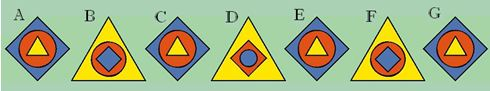1. A

2. B

3. F

4. D

6. What should come next in pattern: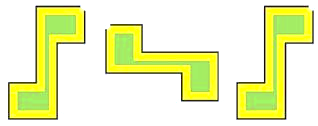1.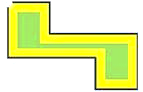2.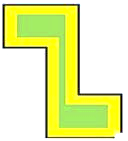3.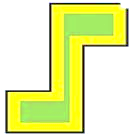4.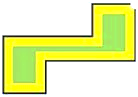7. Complete the pattern: 100, 80, 60, 40, ___, ___

1. 20, 10

2. 30, 20

3. 20, 0

4. 30, 0

8. Match the following:

 (a) AB,cd,DE,fg,_____,_______. (i)  Ii,Kk (b) aA, bB, cC,______,______ (ii) Ee,Ff (c) Aa,Bb,Cc,Dd_____,_____. (iii) dD,eE (d) Aa,Cc,Ee,Gg_____,____. (iv) HI,jk
9. Complete the pattern: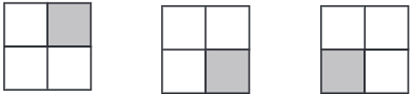10. Using the code A is 1, B is 2, C is 3 and so on, decode the following message:

1. 23   5   12   12
2. 4    15    14    5
11. Study the pattern and fill in the missing numbers:

1.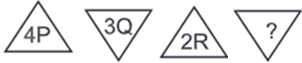2.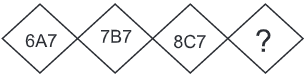12. Examine the number pattern and answer the following questions.
960,860,760,660.....

1. What rule does the pattern follows?
2. Next 5 numbers of patterns?
13. Using the following table write down the symbolic code for given numbers.

 NUMBER 0 1 2 3 4 5 6 7 8 9 SIGN + - * / @ # \$ % & >
1. 263548
2. 007143
14. Complete the pattern.

1. 1, 1, 2, 3, 5, 8, ____,______,______.
2. 1, 2, 4, 7, 11,______,_______,_____.
3. A1000, B2000, C3000, D4000,_______,_______,_______.
4. 0.1, 0.01, 0.001, 0.0001, _________,_________,_________.
15. Write the following message using the code given below.

 ALPHABET A B C D E F G H I J K L M N O P Q R S T U V W X Y Z CODE 1 2 3 4 5 6 7 8 9 10 11 12 13 14 15 16 17 18 19 20 21 22 23 24 25 26
1. VICTORY
2. MYCBSEGUIDE
3. INDIA
4. CRICKET
1. If we change each letter by its next letter e.g we write ‘G’ in place of ‘F’ ‘O’ in place of ‘N’.What was the secret message of XF BSF GSJFOET
2. Observe the number patterns and fill in the blanks:
1 + 2 + 3 = 6
2 + 3 + 4 = 9
3 + 4 + 5 = _______
_______ + 5  + _______  =  _______
_______ +  _______  +  _______ =  _______

CBSE Worksheet-01
Class - IV Mathematics – Patterns and symmetry

Solution

1. Fill in the blanks with suitable words:

1. 54

2. 60 and 64 ( Using rule "add 4"  44 +4 = 48, 48 +4 = 52, 52+4 =56, 56+4 = 60, 60+4 = 64)

3. 88

4. 50, 45, 40, 35 (we start from 50 so 50, 50- 5 = 45, 45-5 = 40, 40-5 = 35)

5. 5. (Because each time 5 is subtracted from the previous number)

2. State whether each of the following statement is True or False:

1. True,  2, 2 + 10 = 12, 12+10 = 22,  22 + 10 = 32

2. True, 10, 20, 30, 40, 50, 60, 70, 80, 90 hence, 9th term is 90.

3. True

4. False, Pattern 10, 15, 20, 25 is starting with 10 and follow "add 5" rule, 10, 10 + 5 = 15, 15 + 5 = 20, 20 + 5 = 25

5. False, 100, 90, 80, 70, 60, 50, 40, 30, 20. Hence, 8th term is 30

3. (d) 25W, 24V
Explanation: It's a pattern of combination of reverse order numbers like 28,27,26, 25, 24 and alphabet are Z, Y, X, W, V.  So next number in series 25W, 24V.
4. (d) 28, 33, 38
Explanation: In given pattern difference between two number is 5. So, next numbers are 23 + 5 = 28, 28 + 5 = 33  and 33 + 5 = 38.
5. (d) D
Explanation: Picture D breaking the rule because in picture D pattern of circle, triangle and quadrilateral is different from others two.
6. (a)Explanation: The positions of pictures are changing from vertical to horizontal directions alternatively. So next picture is horizontal.
7. (c) 20, 0
Explanation: Here numbers decrease by 20. So next two numbers are 40 – 20 = 20 and 20 – 20 = 0.
8. (a) – (iv), (b) – (iii), (c) – (ii), (d) – (i)

9. Every time the figure rotates 90o
So, after third rotation the figure will be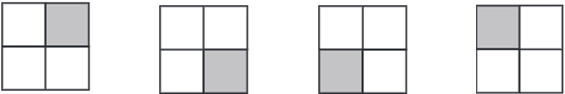1. W  E  L   L
2. D  O  N  E
1. 1S
as we follow the pattern we know that, number is move 1 backward every time and alphabet move 1 forward every time.
2. 9D7
as we follow the pattern we know that, number is move 1 forward every time, alphabet also move 1 forward every time and again number which remain same every time.
10. 960,860,760,660.....
960-100 = 860
860-100 = 760
760-100 = 660
660-100 = 560
560-100 = 460
460-100 = 360
360-100 = 260
260-100 = 160
Next five numbers are 560, 460, 360, 260, 160

11.  NUMBER 0 1 2 3 4 5 6 7 8 9 SIGN + - * / @ # \$ % & >
1. 263548 = *\$/#@&
2. 007143 = ++%-@/
1. 1,1,2,3,5, ____,______,______.
Here, the sum of the two numbers is the next number.
1
1+1 = 2
1+2 = 3
2+3 = 5
3+5 = 8
5+8 = 13
8+13 = 21
1,1,2,3,5,8,13,21
2. 1,2,4,7,11,______,_______,_____.
Add 1 to 1st number  1+ 1 = 2
Add 2 to 2nd number 2+ 2 = 4
Add 3 to 3rd number  4+ 3 = 7
Add 4 to 4th number  7 + 4 = 11
Add 5 to 5th number 11 + 5 = 16
Add 6 to 6th number 16 + 6 = 22
Add 7 to 7th number 22 + 7 = 29
1,2,4,7,11,16,22,29
3. A1000,B2000,C3000,D4000,_______,_______,_______.
A(1000) = A1000
B(1000+1000) = B2000
C(2000+1000) = C3000
D(3000+1000) = D4000
E(4000+1000) = E5000
F(5000+1000) = F6000
G(6000+1000) = G7000
A1000,B2000,C3000,D4000,E5000,F6000,G7000
4. 0.1,0.01,0.001,0.0001,_________,_________,_________.
Increase numbers of zero after decimal point
0.1,0.01,0.001,0.0001,0.00001,0.000001,0.0000001
12.  ALPHABET A B C D E F G H I J K L M N O P Q R S T U V W X Y Z CODE 1 2 3 4 5 6 7 8 9 10 11 12 13 14 15 16 17 18 19 20 21 22 23 24 25 26
1. V I C T O R Y = 23 9 3 20 15 18 25
2. M Y C B S E G U I D E = 13 25 3 2 19 5 7 21 9 4 5
3. INDIA = 9 14 4 9 1
4. CRICKET= 3 18 9 3 11 5 20
13. Here you can see the pattern, first one is adding 1+2+3 and the second one is the next number 2+3+4 and third is 3+4+5 and it goes no and the sum is the multiple of 3 (6,9,12,15,18)

1. The secret message of XF BSF GSJFOET is  "WE ARE FRIENDS"
Hint: X comes after W and F comes after E and so for XF we wrote WE, similarly it's done for the other letters
2. 1 + 2 + 3 = 6
2 + 3 + 4 = 9
3 + 4 + 5 = 12
+ 5 + 6 = 15
5 + 7 = 18